# On a class of quartic Diophantine equations

F. Izadi, M. Baghalaghdam and S. Kosari
Notes on Number Theory and Discrete Mathematics
Print ISSN 1310–5132, Online ISSN 2367–8275
Volume 27, 2021, Number 1, Pages 1—6
DOI: 10.7546/nntdm.2021.27.1.1-6

## Details

### Authors and affiliations

F. IzadiDepartment of Mathematics, Faculty of Science,
Urmia University, Urmia 165-57153, Iran

M. BaghalaghdamDepartment of Mathematics, Faculty of Science,
Azarbaijan Shahid Madani University, Tabriz 53751-71379, Iran

S. KosariInstitute of Computing Science and Technology, Guangzhou University
Guangzhou, 510006, China

### Abstract

In this paper, by using elliptic curves theory, we study the quartic Diophantine equation (DE)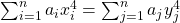, where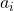andare fixed arbitrary integers. We try to transform this quartic to a cubic elliptic curve of positive rank. We solve the equation for some values ofand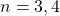, and find infinitely many nontrivial solutions for each case in natural numbers, and show among other things, how some numbers can be written as sums of three, four, or more biquadrates in two different ways. While our method can be used for solving the equation for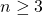, this paper will be restricted to the examples where. Finally, we explain how to solve more general cases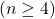without giving concrete examples to case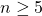.

### Keywords

• Quartic Diophantine equations
• Elliptic curves

• 11D45
• 11D72
• 11D25
• 11G05
• 14H52

### References

1. Bremner, A., Choudhry, A., & Ulas, M. (2014). Constructions of diagonal quartic and sextic surfaces with infinitely many rational points, International Journal of Number Theory, 10(7), 1675–1698.
2. Baghalaghdam, M., & Izadi, F. Is the quartic Diophantine equation A4 + hB4 = C4 + hD4, solvable for any integer h?, submitted.
3. Dickson, L. E. (1934). History of the Theory of Numbers, Vol. II: Diophantine Analysis, G. E. Stechert Co., New York.
4. Elkies, N. (1988). On A4 + B4 + C4 = D4. Mathematics of Computation, 51(184), 825–835.
5. Lander, L. J., & Parkin, T. R. (1966). Counterexamples to Euler’s conjecture on sums of like powers, Bull. Amer. Math. Soc, 72, 1079.
6. Sage software, available from http://sagemath.org.

## Cite this paper

Izadi, F., Baghalaghdam, M., & Kosari, S. (2021). On a class of quartic Diophantine equations. Notes on Number Theory and Discrete Mathematics, 27(1), 1-6, doi: 10.7546/nntdm.2021.27.1.1-6.